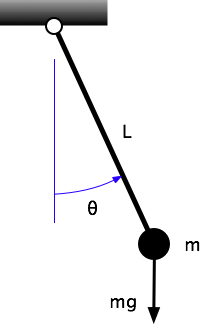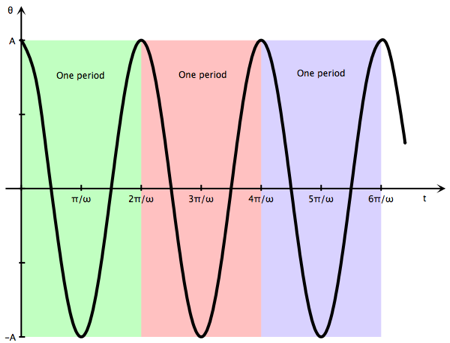# Swingin'

A couple of days ago I saw this poster of time traveler hints via a tweet by @gnomedad. It’s cute, but

• It’s been done better.
• It seems to assume you won’t be traveling back any earlier than the Victorian age. Most of the hints are worthless without an infrastructure developed by the Industrial Revolution1. For example, it’s nice to know about wing sections, but without an engine you’ll never get off the ground.

Anyway, there was one thing on the poster I found interesting.

The exact speed of light in a vacuum is 299,792,458 meters per second. Good to know. A meter is defined in terms of light, but if you can’t measure it accurately, the length of a pendulum that takes one second to swing from end to end will do the trick.

Let’s ignore the odd notion that you’re going to be able to use the speed of light to lay out a line one meter long (no problem, just invent a clock accurate to $$10^{-9}$$ seconds), and focus on that thing about the pendulum. Why would a one-meter pendulum take exactly one second to swing from side to side? I’ve analyzed the behavior of pendulums as both a student and a teacher, and I don’t ever remember running across that fact. But it’s fairly easy to derive from first principles, and since I’m not teaching anymore, I’ll inflict the derivation on you.

Here’s a simple pendulum consisting of a small ball of mass $$m$$ hanging from a pivot point by a string of length $$L$$.The ball is so small (compared to the length of the string) that we don’t have to account for its radius, and the string is so light (compared to the ball) that we don’t have to account for its mass. We’ll call the angle of the string from the vertical $$\theta$$ and construct the equation of motion through Euler’s second law, a rotational analogue of Newton’s second law2:

$\sum M_O = I_O\:\ddot{\theta}$

$$\sum M_O$$ is the sum of all the moments about the pivot point, $$O$$; $$I_O$$ is the mass moment of inertia of the pendulum about the pivot point; and $$\ddot{\theta}$$ is the angular acceleration of the pendulum, the second time derivative of the position angle $$\theta$$. Since we are taking the entire mass of the pendulum to be $$m$$ concentrated at a distance $$L$$ from the pivot,

$I_O = m L^2$

The reaction force at the pivot doesn’t generate a moment about the pivot because its lever arm is zero. Therefore, the only force that contributes to $$\sum M_O$$ is the weight of the pendulum, $$mg$$, where $$g$$ is the acceleration due to gravity. Its lever arm is $$L \sin \theta$$, so

$-m g L \sin \theta = m L^2 \ddot{\theta}$

where the negative sign indicates that the moment is in the opposite direction of $$\theta$$. Canceling the common terms and rearranging, we get

$\ddot{\theta} + \frac{g}{L} \sin \theta = 0$

This is a rather nasty differential equation because of the sine term. If we assume the swing angle stays relatively small throughout the motion, we can make the further simplification, $$\sin \theta \approx \theta$$. How good is this approximation? It’s easy to make up a little table and check.

θ (deg) θ (rad) sin(θ) error
0.00 0.0000 0.0000
1.00 0.0175 0.0175 0.01%
2.00 0.0349 0.0349 0.02%
3.00 0.0524 0.0523 0.05%
4.00 0.0698 0.0698 0.08%
5.00 0.0873 0.0872 0.13%
6.00 0.1047 0.1045 0.18%
7.00 0.1222 0.1219 0.25%
8.00 0.1396 0.1392 0.33%
9.00 0.1571 0.1564 0.41%
10.00 0.1745 0.1736 0.51%
11.00 0.1920 0.1908 0.62%
12.00 0.2094 0.2079 0.73%
13.00 0.2269 0.2250 0.86%
14.00 0.2443 0.2419 1.00%
15.00 0.2618 0.2588 1.15%

So the error is less than 1% if the pendulum doesn’t swing more than 14° from vertical and less than 0.5% if it swings no more than 10° from vertical. Not bad.

The small angle approximation leads to

$\ddot{\theta} + \frac{g}{L} \theta = 0$

This is a second order linear differential equation with constant coefficients, which is, as these things go, quite easy to solve. The solution will have the form

$\theta = A \cos\omega t + B \sin \omega t$

where $$\omega = \sqrt{g/L}$$ is the the circular frequency of the oscillation, and $$A$$ and $$B$$ depend on the initial conditions, the angle and angular velocity of the pendulum at time zero. For a pendulum pulled to the side and released from rest, the solution takes the form

$\theta = A \cos \omega t$

with $$A$$ being the amplitude of the oscillation, the largest value of the swing angle. The periodic motion of the pendulum—left to right to left to right, and so on—is reflected in the periodic nature of the cosine function.In our solution, the pendulum never slows down or stops because we haven’t modeled air resistance or friction at the pivot.

The period of the pendulum—one back-and-forth cycle—is

$\frac{2 \pi}{\omega} = 2 \pi \sqrt{\frac{L}{g}}$

So the time it takes to swing from one side to the other is half that:

$\pi \sqrt{\frac{L}{g}} = \sqrt{\frac{\pi^2 L}{g}}$

In SI units, $$g = 9.81\:\mathrm{m/s^2}$$. For a pendulum one meter long, the time to swing from one side to the other is

$\sqrt{\frac{\pi^2 \cdot 1 \mathrm{m}}{9.81\:\mathrm{m/s^2}}} = 1.003\:\mathrm{s}$

I think it’s fair to say that we can ignore the 3 millisecond difference and declare that a 1-meter pendulum has a half-period of 1 second.

This nice result comes about because the gravitational acceleration on the surface of the Earth in $$m/s^2$$ is just about equal to $$\pi^2$$. In fact, there was a time when the meter was going to be defined in terms of this pendulum length, but that definition lost out to the meridonal definition.

Pi is a universal constant because it’s based on mathematics alone, but $$g$$ isn’t; so if your time machine also transports you to another planet, don’t expect this trick to work.

1. Yes, I know it’s just for fun, but much of the fun of science fiction lies in being as realistic as possible after accepting one or two unrealistic premises.

2. As always, the equations in this post are formatted by jsMath, a wonderful JavaScript library for LaTeX rendering written by Davide Cervone. I prefer jsMath to ASCIIMathML because it works in all browsers, not just those with MathML support. You can fiddle with the jsMath settings by clicking the little jsMath button that should be in the lower right corner of your window. To get the most out of any browser-rendered math—jsMath or ASCIIMath—you should have a set of TeX fonts installed on your computer.

If you’re reading this in an RSS reader, the equations won’t render and you’ll just see the raw LaTeX. I’m looking into fixing that.